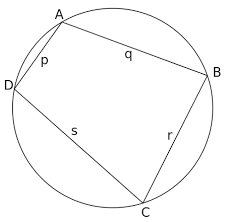Given is a quadrilateral ABCD inscribed in a circle, with the diagonal AC being the circle's diameter. The distance between point B and the diameter is 15 cm, and between point D and the diameter is 18 cm. Calculate the radius of the circle and the perimeter of the quadrilateral ABCD.

r =  1 cm
o =  0 cm

### Step-by-step explanation:Did you find an error or inaccuracy? Feel free to write us. Thank you!

Tips for related online calculators

#### Grade of the word problem:

We encourage you to watch this tutorial video on this math problem: